# Section 2 2 Linear Equations 4 Linear equations

• Slides: 19Section 2 -2 : Linear Equations 4 Linear equations graph as lines. 4 Standard form: Ax + By = C, where A, B, & C are real numbers. (A & B cannot both be 0). 4 The key to standard form is that both variables are on the same side.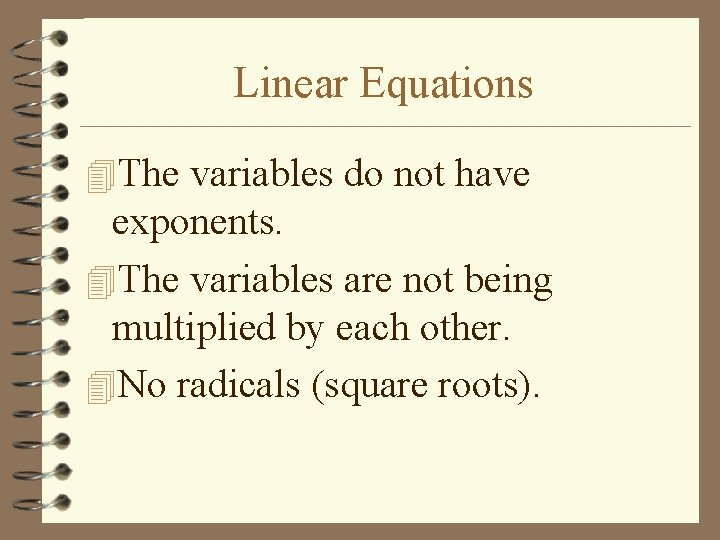Linear Equations 4 The variables do not have exponents. 4 The variables are not being multiplied by each other. 4 No radicals (square roots).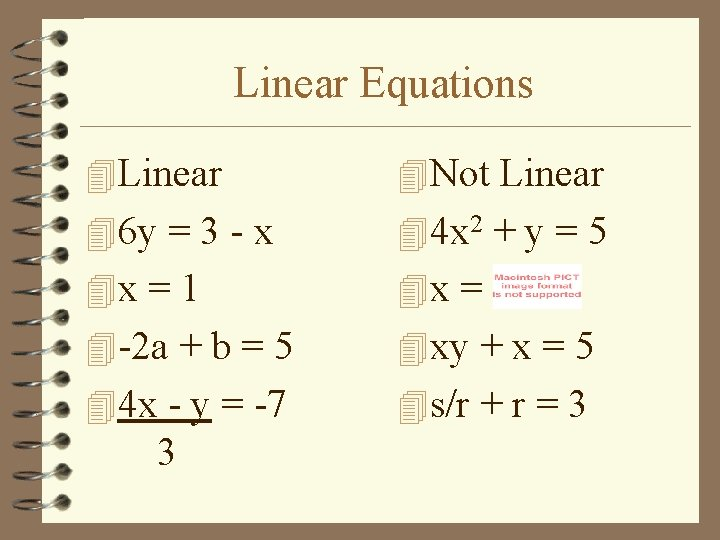Linear Equations 4 Linear 4 Not Linear 46 y = 3 - x 44 x 2 + y = 5 4 x = 1 4 x = 4 -2 a + b = 5 4 xy + x = 5 44 x - y = -7 4 s/r + r = 3 3Linear Equations 4 Linear Functions: linear equations that are functions (no value of the domain is repeated). 4 Usually in the form f(x) = mx + b. (hopefully you remember y =mx+b which is slope-intercept form of an equation. m = slope, b = y-int. )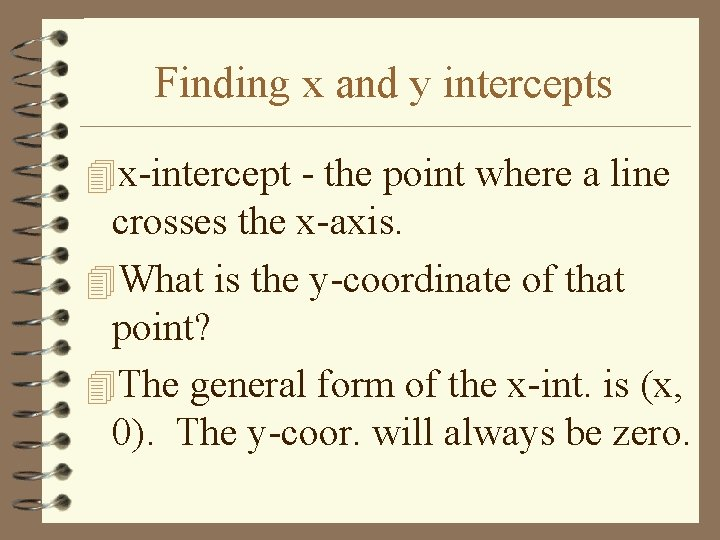Finding x and y intercepts 4 x-intercept - the point where a line crosses the x-axis. 4 What is the y-coordinate of that point? 4 The general form of the x-int. is (x, 0). The y-coor. will always be zero.Finding x and y intercepts 4 y-intercept - the point where a line crosses the y-axis. 4 What is the x-coordinate of that point? 4 The general form of the y-int. is (0, y). The x-coor. will always be zero.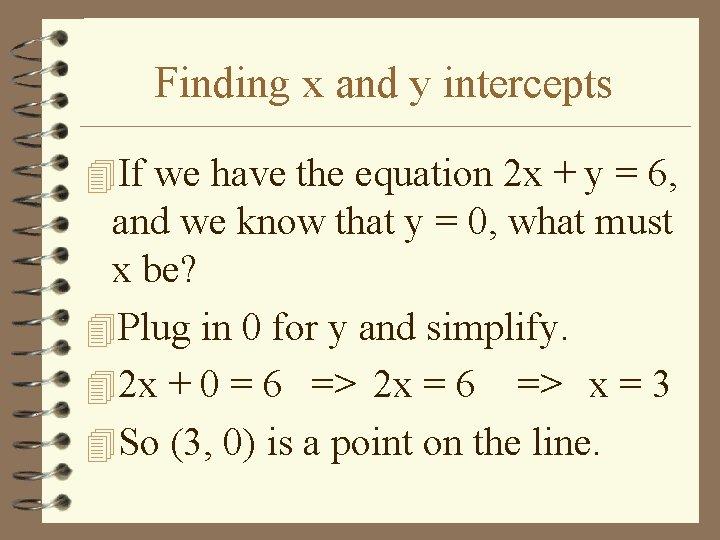Finding x and y intercepts 4 If we have the equation 2 x + y = 6, and we know that y = 0, what must x be? 4 Plug in 0 for y and simplify. 42 x + 0 = 6 => 2 x = 6 => x = 3 4 So (3, 0) is a point on the line.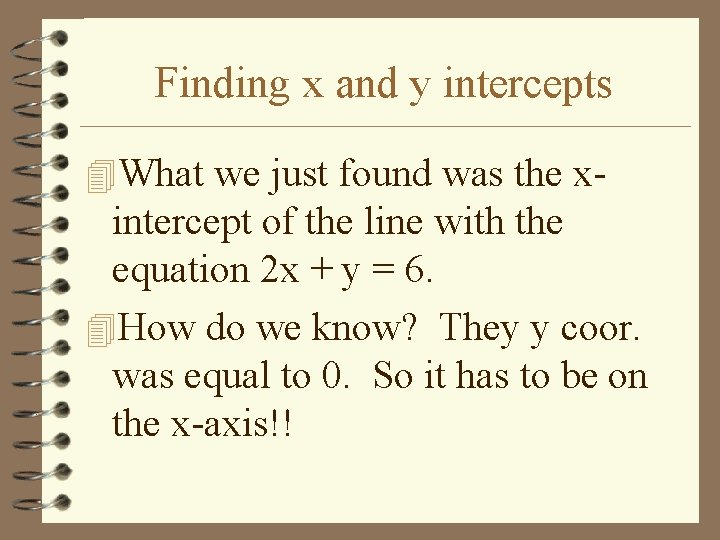Finding x and y intercepts 4 What we just found was the x- intercept of the line with the equation 2 x + y = 6. 4 How do we know? They y coor. was equal to 0. So it has to be on the x-axis!!Finding x and y intercepts 4 To find the x-intercept, plug in 0 for y. 4 To find the y-intercept, plug in 0 for x.Find the x and y intercept x = 4 y - 5 4 x-intercept: 4 y-intercept: 4 Plug in y = 0 4 Plug in x = 0 4 x = 4(0) - 5 40 = 4 y - 5 4 x = 0 - 5 45 = 4 y 4 x = -5 45/4 = y 4(-5, 0) is the x 4(0, 5/4) is the y -int.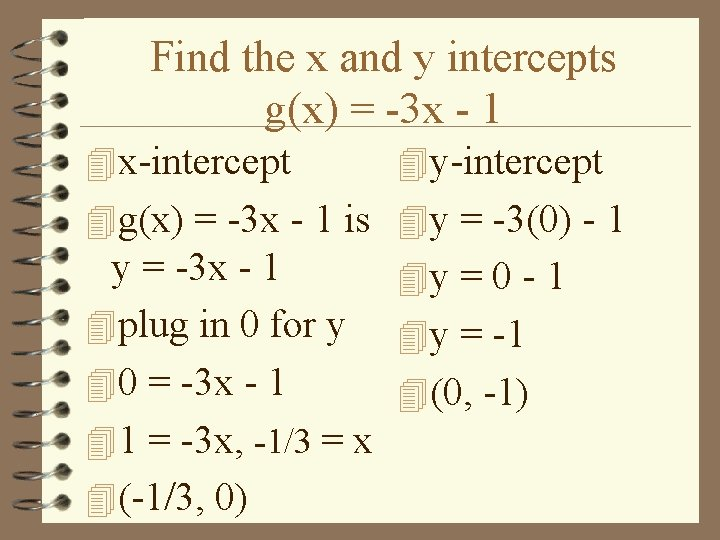Find the x and y intercepts g(x) = -3 x - 1 4 x-intercept 4 y-intercept 4 g(x) = -3 x - 1 is 4 y = -3(0) - 1 y = -3 x - 1 4 y = 0 - 1 4 plug in 0 for y 4 y = -1 40 = -3 x - 1 4(0, -1) 41 = -3 x, -1/3 = x 4(-1/3, 0)find the x and y intercepts 6 x - 3 y =-18 4 x-intercept 4 y-intercept 46 x - 3(0) = -18 46(0) - 3 y = -18 46 x - 0 = -18 40 - 3 y = -18 46 x = -18 4 -3 y = -18 4 x = -3 4 y = 6 4(-3, 0) 4(0, 6)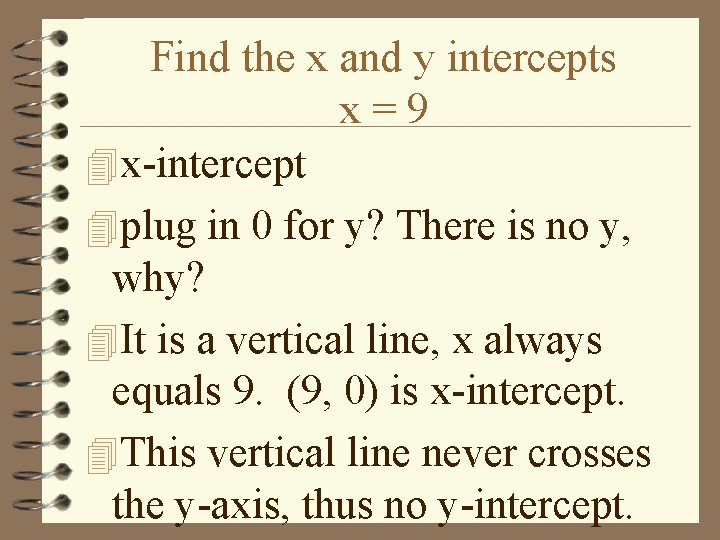Find the x and y intercepts x=9 4 x-intercept 4 plug in 0 for y? There is no y, why? 4 It is a vertical line, x always equals 9. (9, 0) is x-intercept. 4 This vertical line never crosses the y-axis, thus no y-intercept.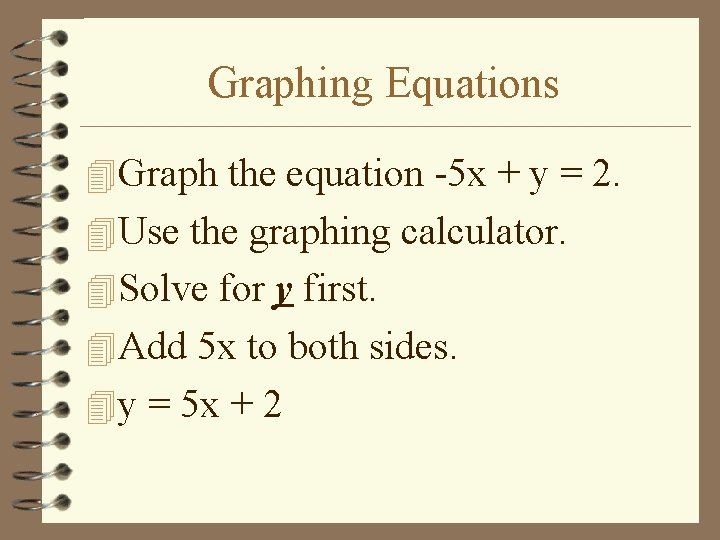Graphing Equations 4 Graph the equation -5 x + y = 2. 4 Use the graphing calculator. 4 Solve for y first. 4 Add 5 x to both sides. 4 y = 5 x + 2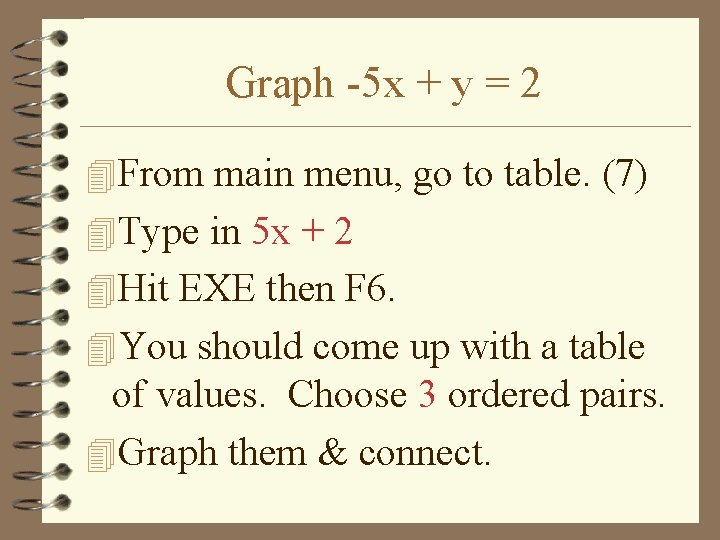Graph -5 x + y = 2 4 From main menu, go to table. (7) 4 Type in 5 x + 2 4 Hit EXE then F 6. 4 You should come up with a table of values. Choose 3 ordered pairs. 4 Graph them & connect.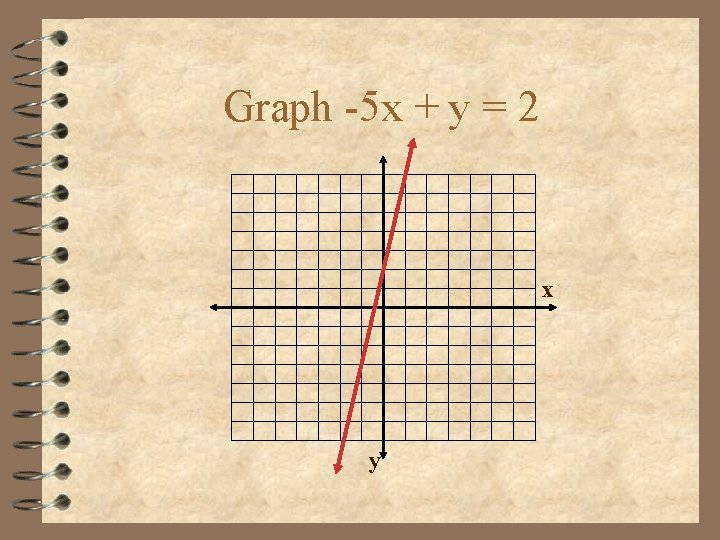Graph -5 x + y = 2 x yGraph 4 x - 3 y = 12 4 Solve for y. Subtract 4 x from both sides. 4 -3 y = -4 x + 12. Divide by -3. 4 y = -4 x/-3 + 12/-3. Simplify. 4 y = x - 4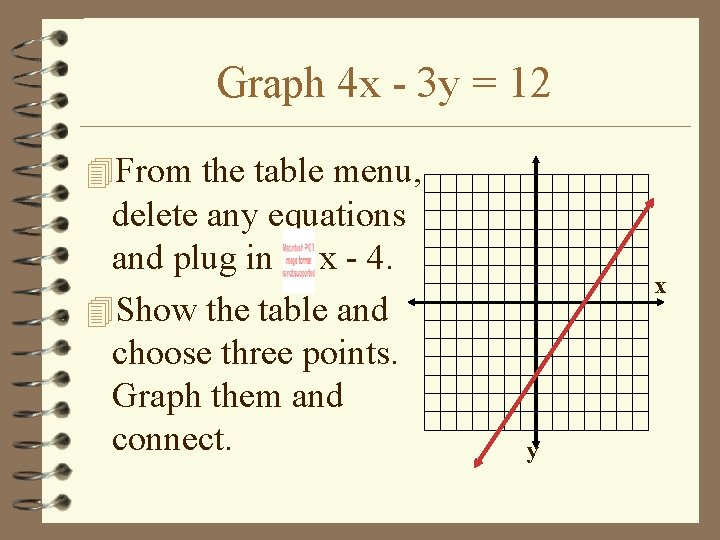Graph 4 x - 3 y = 12 4 From the table menu, delete any equations and plug in x - 4. 4 Show the table and choose three points. Graph them and connect. x yAssignment: Section 2 -2 Wkst 2. 2 (from share folder)